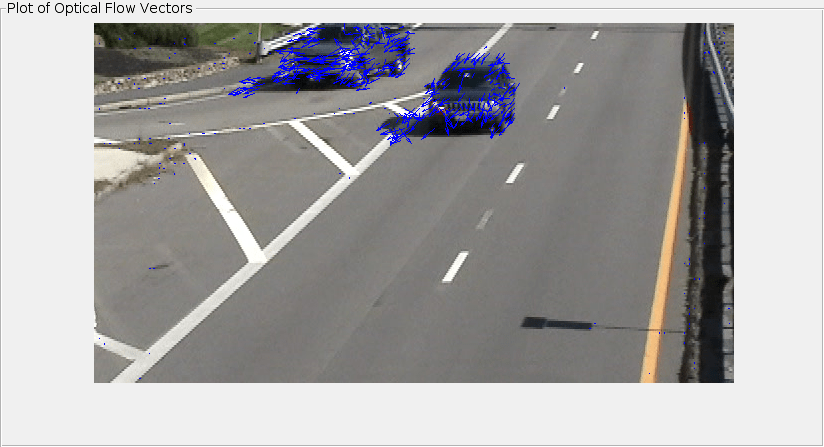# opticalFlowLKDoG

Object for estimating optical flow using Lucas-Kanade derivative of Gaussian method

## Description

Create an optical flow object for estimating the direction and speed of moving objects using the Lucas-Kanade derivative of Gaussian (DoG) method. Use the object function `estimateFlow` to estimate the optical flow vectors. Using the `reset` object function, you can reset the internal state of the optical flow object.

## Creation

### Syntax

``opticFlow = opticalFlowLKDoG``
``opticFlow = opticalFlowLKDoG(Name,Value)``

### Description

````opticFlow = opticalFlowLKDoG` returns an optical flow object that you can use to estimate the direction and speed of the moving objects in a video. The optical flow is estimated using the Lucas-Kanade derivative of Gaussian (DoG) method.```

example

````opticFlow = opticalFlowLKDoG(Name,Value)` returns an optical flow object with properties specified as one or more `Name,Value` pair arguments. Any unspecified properties have default values. Enclose each property name in quotes.For example, `opticalFlowLKDoG('NumFrames',3)````

## Properties

expand all

Number of buffered frames for temporal smoothing, specified as a positive integer-valued scalar. As you increase this number, the optical flow estimation method becomes less robust to abrupt changes in the trajectory of the moving objects. The amount of delay in flow estimation depends on the value of `NumFrames`. The output flow corresponds to the image at tflow = tcurrent − 0.5(NumFrames-1), where tcurrent is the time of the current image.

Standard deviation for image smoothing filter, specified as a positive scalar.

Standard deviation for gradient smoothing filter, specified as a positive scalar.

Threshold for noise reduction, specified as a positive scalar. As you increase this number, the movement of the objects has less impact on optical flow calculation.

## Object Functions

 `estimateFlow` Estimate optical flow `reset` Reset the internal state of the optical flow estimation object

## Examples

collapse all

Read a video file. Specify the timestamp of the frame to be read.

`vidReader = VideoReader('visiontraffic.avi','CurrentTime',11);`

Create an optical flow object for estimating the optical flow using Lucas-Kanade DoG method. Specify the threshold for noise reduction. The output is an optical flow object specifying the optical flow estimation method and its properties.

`opticFlow = opticalFlowLKDoG('NoiseThreshold',0.0005)`
```opticFlow = opticalFlowLKDoG with properties: NumFrames: 3 ImageFilterSigma: 1.5000 GradientFilterSigma: 1 NoiseThreshold: 5.0000e-04 ```

Create a custom figure window to visualize the optical flow vectors.

```h = figure; movegui(h); hViewPanel = uipanel(h,'Position',[0 0 1 1],'Title','Plot of Optical Flow Vectors'); hPlot = axes(hViewPanel);```

Read the image frames and convert to grayscale images. Estimate the optical flow from consecutive image frames. Display the current image frame and plot the optical flow vectors as quiver plot.

```while hasFrame(vidReader) frameRGB = readFrame(vidReader); frameGray = im2gray(frameRGB); flow = estimateFlow(opticFlow,frameGray); imshow(frameRGB) hold on plot(flow,'DecimationFactor',[5 5],'ScaleFactor',35,'Parent',hPlot); hold off pause(10^-3) end```## Algorithms

expand all

To compute the optical flow between two images, you must solve this optical flow constraint equation:

`${I}_{x}u+{I}_{y}v+{I}_{t}=0$`

.

• ${I}_{x}$, ${I}_{y}$, and ${I}_{t}$ are the spatiotemporal image brightness derivatives.

• u is the horizontal optical flow.

• v is the vertical optical flow.

 Barron, J. L., D. J. Fleet, S. S. Beauchemin, and T. A. Burkitt. “ Performance of optical flow techniques.” In Proceedings of the IEEE Conference on Computer Vision and Pattern Recognition (CVPR),236-242. Champaign, IL: CVPR, 1992.